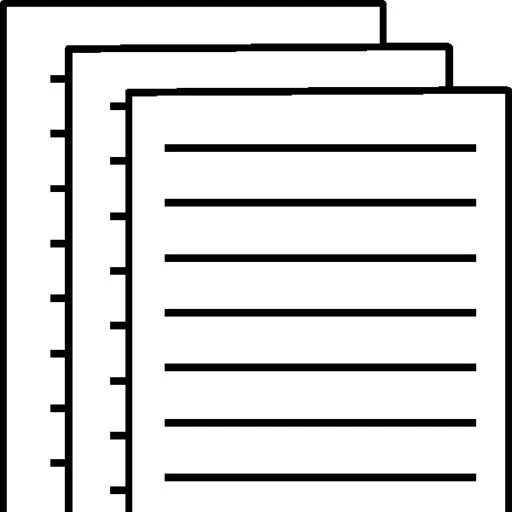## Domains of Rational Expressions

Consider the rational expression:

To factor the expression, factor the numerator and denominator completely.

Given rational expression

Rewrite the numerator

Use

So, the factored expression is.

The domain of an expression is the set of all real numbers for which the expression is defined.

The rational expression is undefined when the denominator is zero, because division by zero is undefined. To find the domain of rational expression set denominator equal to zero and exclude those value from the domain.

So, the excluded value is.

Therefore, the domain of rational expression is.

Consider the rational expression:

To factor the expression, factor the numerator and denominator completely.

Given rational expression

Break middle term of numerator

Group pairs

Factor each pair

So, the factored expression is.

The rational expression is undefined when the denominator is zero, because division by zero is undefined. To find the domain of rational expression set denominator equal to zero and exclude those value from the domain.

So, the excluded value isand.

Therefore, the domain of rational expression is.

To view and download a complete answer, scroll down to the bottom to pay